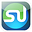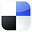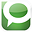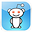# ANOVA Test Done in Excel For Better PPC Marketing

If you have ever run a pay-per-click campaign, you’ve probably wondered which factors really made a difference in the click-through rates. Are the headlines really making a difference in CTR? Does the ad text have any affect on CTR? What about the interaction between ad text and headline?

The good news is that there is a statistical tool in Excel designed just for a test like that. The tool is called ANOVA: Two Factor With Replication. Here is a video which shows exactly how to perform this test.

Step-By-Step Video On How To Increase Your Click-Through Rate With ANOVA in Excel

We Used ANOVA To Test for the Following

To sum up this test, we are determining whether one or both of two factors (headline and ad text) and/or the interaction between the two affected click-through rate. We are replicating the same test in two environments: on Google and also on Yahoo pay-per-click advertising programs. The replication creates the opportunity to evaluate of whether interaction between Headline and Ad Text affected CTR.

What Is ANOVA?

ANOVA, Analysis of Variance, is a test to determine if three or more different methods or treatments have the same effect on a population. The basic test of ANOVA is the Null Hypothesis, which states that varying a factor has no effect on the output. Each factor and the interaction between the two factors has its own separate Null Hypothesis.

The Null Hypothesis connected with the headlines states that choice of headlines has no effect on the measured output, the click-through rate. The null Hypothesis connected with the ad text states that choice of headlines has no effect on the output. The Null Hypothesis connected with the interaction between headline and ad text states that this interaction has no effect on the click-through rate.

Here's How We Ran Our ANOVA Test

The test was run as follows: 3 headlines and three sets of ad text were created. Altogether there were 9 possible combinations of headline / ad text. All 9 ads were run for approximately equal number of times on both the Google and Yahoo paid search networks. The Excel tool: ANOVA: Two Factor With Replication will then be run on the results to determine with at least 95% certainty whether headline, ad text, and/or their interaction had an effect on the click-through rate.

This is how the data needs to be organized on the Excel spread sheet. The yellow-highlighted cells are the input data for the Excel ANOVA.

Click On Image To See Enlarged View

The above video shows the Click-Through Rates results and also how to insert the data into Excel so the ANOVA test can be run on the data.

How To Interpret the ANOVA Output From Excel

Click On Image To See Enlarged View

The Excel output of the test is fairly simple to interpret. The linked video above shows exactly how that is done. In a nutshell, a factor (headline, ad text, or interaction between the two) is said to affect the output (click-through rate) if the P Value associated with that factor is less than the alpha. The alpha is derived from the level of certainty required. Alpha = 100% - Level of Certainty. For example, if a 95% level of certainty is required, then the alpha = 0.05 (100% - 95% = 5% or 0.05). The P Value associated with a factor equals the probability that the output occurred by chance.

The P Value Rule

As a general rule, if the P Value of a factor is less that the alpha, then we can reject that factor’s Null Hypothesis, which states that varying the factor has no effect on the output.

On the other hand, if the P Value of a factor is greater than the alpha, we cannot reject that factor’s Null Hypothesis. We cannot say that varying the factor had an affect on the output.
In this case, we are able to state with 95% certainty that the headlines and interaction affected the output but not the ad text.

Excel Master Series Blog Directory

Statistical Topics and Articles In Each Topic

• Histograms in Excel
• Bar Chart in Excel
• Combinations & Permutations in Excel
• Normal Distribution in Excel
• t-Distribution in Excel
• Binomial Distribution in Excel
• z-Tests in Excel
• t-Tests in Excel
• Hypothesis Tests of Proportion in Excel
• Chi-Square Independence Tests in Excel
• Chi-Square Goodness-Of-Fit Tests in Excel
• F Tests in Excel
• Correlation in Excel
• Pearson Correlation in Excel
• Spearman Correlation in Excel
• Confidence Intervals in Excel
• Simple Linear Regression in Excel
• Multiple Linear Regression in Excel
• Logistic Regression in Excel
• Single-Factor ANOVA in Excel
• Two-Factor ANOVA With Replication in Excel
• Two-Factor ANOVA Without Replication in Excel
• Creating Interactive Graphs of Statistical Distributions in Excel
• Solving Problems With Other Distributions in Excel
• Optimization With Excel Solver
• Chi-Square Population Variance Test in Excel
• Analyzing Data With Pivot Tables
• SEO Functions in Excel
• Time Series Analysis in Excel

Click On Image To See Enlarged View

If You Like This, Then Share It...Excel Master Series Blog Directory

Statistical Topics and Articles In Each Topic

• Histograms in Excel
• Bar Chart in Excel
• Combinations & Permutations in Excel
• Normal Distribution in Excel
• t-Distribution in Excel
• Binomial Distribution in Excel
• z-Tests in Excel
• t-Tests in Excel
• Hypothesis Tests of Proportion in Excel
• Chi-Square Independence Tests in Excel
• Chi-Square Goodness-Of-Fit Tests in Excel
• F Tests in Excel
• Correlation in Excel
• Pearson Correlation in Excel
• Spearman Correlation in Excel
• Confidence Intervals in Excel
• Simple Linear Regression in Excel
• Multiple Linear Regression in Excel
• Logistic Regression in Excel
• Single-Factor ANOVA in Excel
• Two-Factor ANOVA With Replication in Excel
• Two-Factor ANOVA Without Replication in Excel
• Creating Interactive Graphs of Statistical Distributions in Excel
• Solving Problems With Other Distributions in Excel
• Optimization With Excel Solver
• Chi-Square Population Variance Test in Excel
• Analyzing Data With Pivot Tables
• SEO Functions in Excel
• Time Series Analysis in Excel

#### 1 comment:

1.